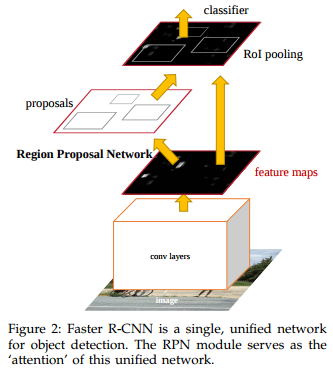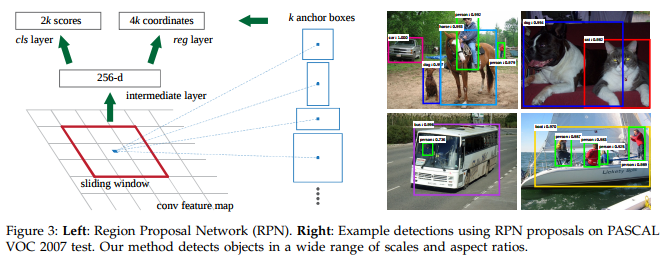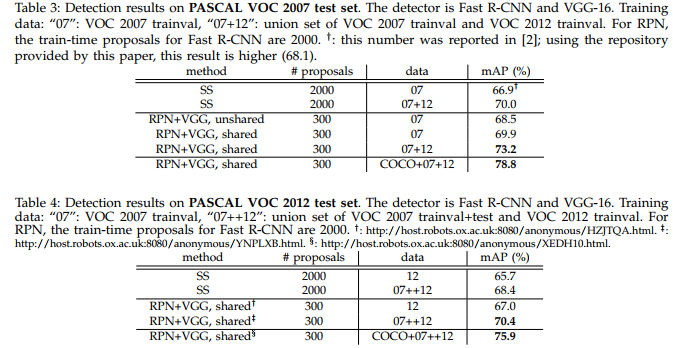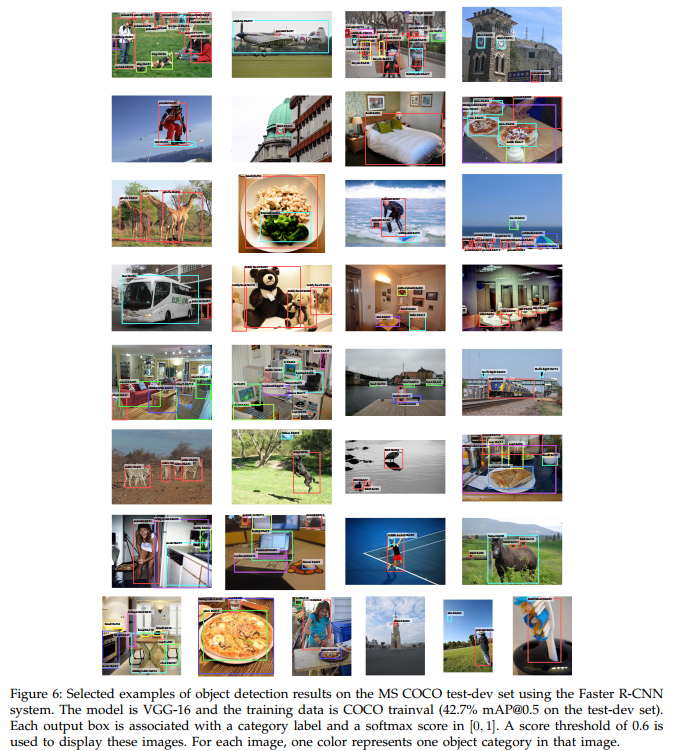This is an effort to improve R-CNN and Fast-CNN. The main improvements are :

• The author uses a Region Proposal Network (RPN) that proposes boxes out of a given feature map which allows end-to-end training (previous methods used a third party proposal method).

• They use multi-resolution anchor boxes## Conv layers

They use VGG-16 and the feature maps fed to the RPN are those in layer 5.

## Region Proposal Network

The RPN shares the same features maps than the Faster RCNN network to allow end-to-end training. To generate region proposals, they slide a small network over the convolutional feature map returned by the last shared convolutional layer (in their implementation, layer 5 of VGG-16). This small network takes as input an $$n\times n$$ spatial window of the input convolutional feature map and output a box-regression layer (reg) and a box-classification layer (cls) (c.f.Figure 3). This architecture is implemented with an $$n\times n$$ convolutional layer followed by two sibling $1 \times 1$ convolutional layers## Loss function

The loss function is the sum of a cross-entropy for the labels and a regression loss between the predicted bounding box and the groundtruth boxes (1 box = 4 coordinates).

## Results

FasterRNN gets respectively a mean AveragePrecision (mAP) of 78.8 and 75.9 on PASCAL VOC 2007 and 2012.## Other stuff

Code from the authors can be found here